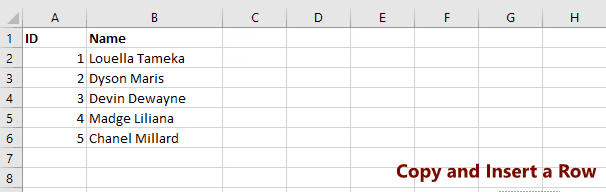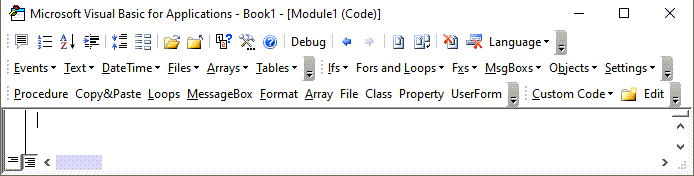# VBA Copy / Paste Rows & Columns

This tutorial will teach you how to copy (or cut) entire Rows or Columns using VBA. We cover copying and pasting ranges of cells in another article.

First we will show you how to paste or insert copied rows/columns and discuss the differences.  Then we will show you all of the different ways to references rows and columns when copying or cutting.

## Rows & Columns – Paste vs. Insert

When pasting rows and columns you have two options:  You can paste over the existing row (or column) or you can insert a new row (or column).

Let’s look at the difference…### Copy & Paste Over Existing Row / Column

This will copy row 1 and paste it into the existing row 5:

``Range("1:1").Copy Range("5:5")``

This will copy column C and paste it into column E:

``Range("C:C").Copy Range("E:E")``

### Copy & Insert Row / Column

Instead you can insert the copied row or column and shift the existing rows or columns to make room.

This will copy row 1 and insert it into row 5, shifting the existing rows down:

``````Range("1:1").Copy
Range("5:5").Insert``````

This will copy column C and insert it into column E, shifting the existing columns to the right:

``````Range("C:C").Copy
Range("E:E").Insert``````

## Copy Entire Row

Below we will show you several ways to copy row 1 and paste into row 5.

``Range("1:1").Copy Range("5:5")``
``Range("A1").EntireRow.Copy Range("A5")``
``Rows(1).Copy Rows(5)``

### Cut and Paste Rows

Simply use Cut instead of Copy to cut and paste rows:

``Rows(1).Cut Rows(5)``

### Copy Multiple Rows

Here are examples of copying multiple rows at once:

``Range("5:7").Copy Range("10:13")``
``Range("A5:A7").EntireRow.Copy Range("A10:A13")``
``Rows("5:7").Copy Rows("10:13")``

Stop searching for VBA code online. Learn more about AutoMacro - A VBA Code Builder that allows beginners to code procedures from scratch with minimal coding knowledge and with many time-saving features for all users!## Copy Entire Column

You can copy entire columns similarily to copying entire rows:

``Range("C:C").Copy Range("E:E")``
``Range("C1").EntireColumn.Copy Range("C1").EntireColumn``
``Columns(3).Copy Range(5)``

### Cut and Paste Columns

Simply use Cut instead of Copy to cut and paste columns:

``Range("C:C").Cut Range("E:E")``

### Copy Multiple Columns

Here are examples of copying multiple columns at once:

``Range("C:E").Copy Range("G:I")``
``Range("C1:E1").EntireColumn.Copy Range("G1:I1")``
``Columns("3:5").Copy Columns("7:9")``

VBA Programming | Code Generator does work for you!

## Copy Rows or Columns to Another Sheet

To copy to another sheet, simply use the Sheet Object:

``Sheets("sheet1").Range("C:E").Copy Sheets("sheet2").Range("G:I")``

### Cut Rows or Columns to Another Sheet

You can use the exact same technique to cut and paste rows or columns to another sheet.

``Sheets("sheet1").Range("C:E").Cut Sheets("sheet2").Range("G:I")``